For a full list of BASHing data blog posts see the index page.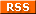# Updating a file from a lookup table

Doing table edits from a lookup table is easy with AWK. Here's tab-separated "fileA":

 Fld1 Fld2 Fld3 Fld4 ooo fff ccc zzz ppp ggg bbb xxx mmm iii aaa www nnn hhh aaa yyy mmm ggg ccc yyy ooo iii ccc xxx nnn hhh bbb www ppp fff aaa zzz

I want to modify the aaa, bbb and ccc entries in field 3 of "fileA" using the lookup table "lookup":

 aaa 111 bbb 222 ccc 333

Three ways to modify might be to replace the aaa, bbb and ccc entries with their lookup numbers, append the numbers within field 3, or insert a new field with the numbers. In all three cases I'll build an array "a" from the lookup table, then move to "fileA" using the FNR==NR trick explained here.

Replace

awk -F"\t" 'FNR==NR {a[\$1]=\$2; next} FNR>1 {\$3=a[\$3]} 1' OFS="\t" lookup fileA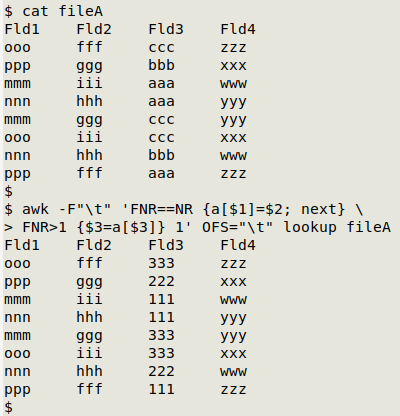Because I'm rebuilding the table, I have to tell AWK not only what the input field separator is (a tab), but also what the field separator will be in the output table (I want it to be a tab, again). There are several ways to do this. Here are three, and for this demo I've chosen the third method:

awk 'BEGIN {FS=OFS="\t"} [command]' lookup fileA
awk -v FS="\t" -v OFS="\t" [command]' lookup fileA
awk -F"\t" [command]' OFS="\t" lookup fileA

When processing the "lookup" file, AWK builds an array "a" where the index strings are the letter entries in the first field (aaa, bbb and ccc), and the value strings corresponding to those index strings are the number entries in the second field (111, 222 and 333): a[\$1]=\$2.

AWK prints all lines in "fileA" because of the "1" at the end of the command. "1" is a condition, and if it's met, AWK does its default action, which is to print the line. The condition "1" is always true (1 always equals 1), so all lines are printed, including the header.

However, before AWK got to the part of the command with condition "1", it dealt with lines matching the condition FNR>1, meaning any line after the header line in "fileA". In those lines, AWK is instructed to redefine the entry in field 3 as the value string from array "a" that corresponds to the field 3 entry: \$3=a[\$3].

Append. This time I'll just add the lookup numbers to the field 3 entries, separating letters and numbers with a hyphen:

awk -F"\t" 'FNR==NR {a[\$1]=\$2; next} FNR>1 {\$3=\$3"-"a[\$3]} 1' OFS="\t" lookup fileAField 3 has been redefined as the original entry, a hyphen, and the lookup number: \$3=\$3"-"a[\$3]

Insert. Here I'm inserting the lookup numbers in a new field just after field 3:

awk -F"\t" 'FNR==NR {a[\$1]=\$2; next} FNR==1 {\$3=\$3 FS "Fld3a"} FNR>1 {\$3=\$3 FS a[\$3]} 1' OFS="\t" lookup fileA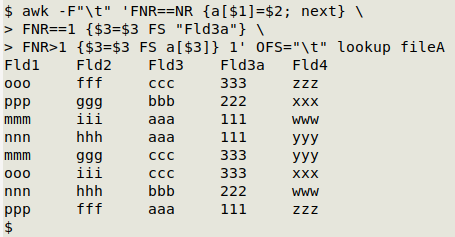The new field needs a name, so I've redefined field 3 in the header line (FNR==1) as field 3, a tab, and "Fld3a" (\$3=\$3 FS "Fld3a"; FS is the field separator defined earlier with -F"\t").

In the following lines (FNR>1), field 3 is redefined as field 3, a tab, and the lookup number corresponding to the entry in field 3 (\$3=\$3 FS a[\$3]).

But what if there's no match? The lookup table might not contain all the different entries in field 3. Maybe one of them is ddd and another is the data entry error a aa, as below in "fileB":

 Fld1 Fld2 Fld3 Fld4 ooo fff ccc zzz ppp ggg bbb xxx mmm iii aaa www nnn hhh ddd yyy mmm ggg ccc yyy ooo iii ccc xxx nnn hhh bbb www ppp fff a aa zzz

If I run the "replace" command on "fileB", the field 3 entries will be blank because ddd and a aa aren't in the lookup table: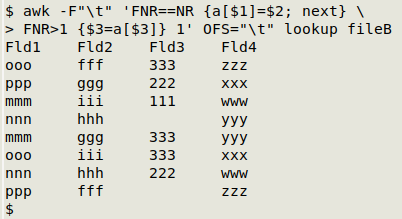It would be nice to have something other than blanks there, maybe an error message like "NOPE". To add this I can use a ternary expression

(Is X true) ? (then do A) : (otherwise do B)

And in this case:

awk -F"\t" 'FNR==NR {a[\$1]=\$2; next} FNR>1 {a[\$3] ? (\$3=a[\$3]) : (\$3="NOPE")} 1' OFS="\t" lookup fileB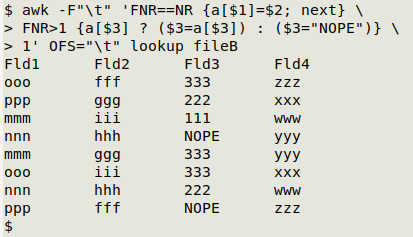The ternary expression asks "Does a[\$3] exist? In other words, is there a value string in the array "a" corresponding to the index string in field 3? If so, the field 3 entry should be a[\$3]. Otherwise, it should be "NOPE". Or a little more informatively:

awk -F"\t" 'FNR==NR {a[\$1]=\$2; next} FNR>1 {a[\$3] ? (\$3=a[\$3]) : (\$3=\$3" not found")} 1' OFS="\t" lookup fileB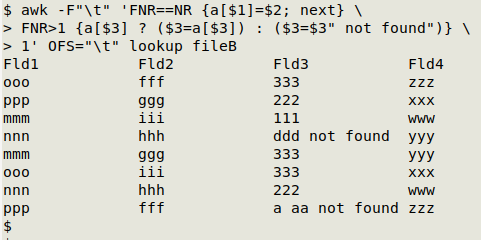But what if you only want to replace with a lookup number in field 3 if there's a "g" in field 2?

No problem, just add that to the condition for replacement:

awk -F"\t" 'FNR==NR {a[\$1]=\$2; next} FNR>1 && \$2 ~ /g/ {\$3=a[\$3]} 1' OFS="\t" lookup fileAif there's a "g" in field 2 can be coded in AWK as \$2 ~ /g/, meaning the field 2 entry matches the regular expression "g".

Last update: 2020-11-11
The blog posts on this website are licensed under a